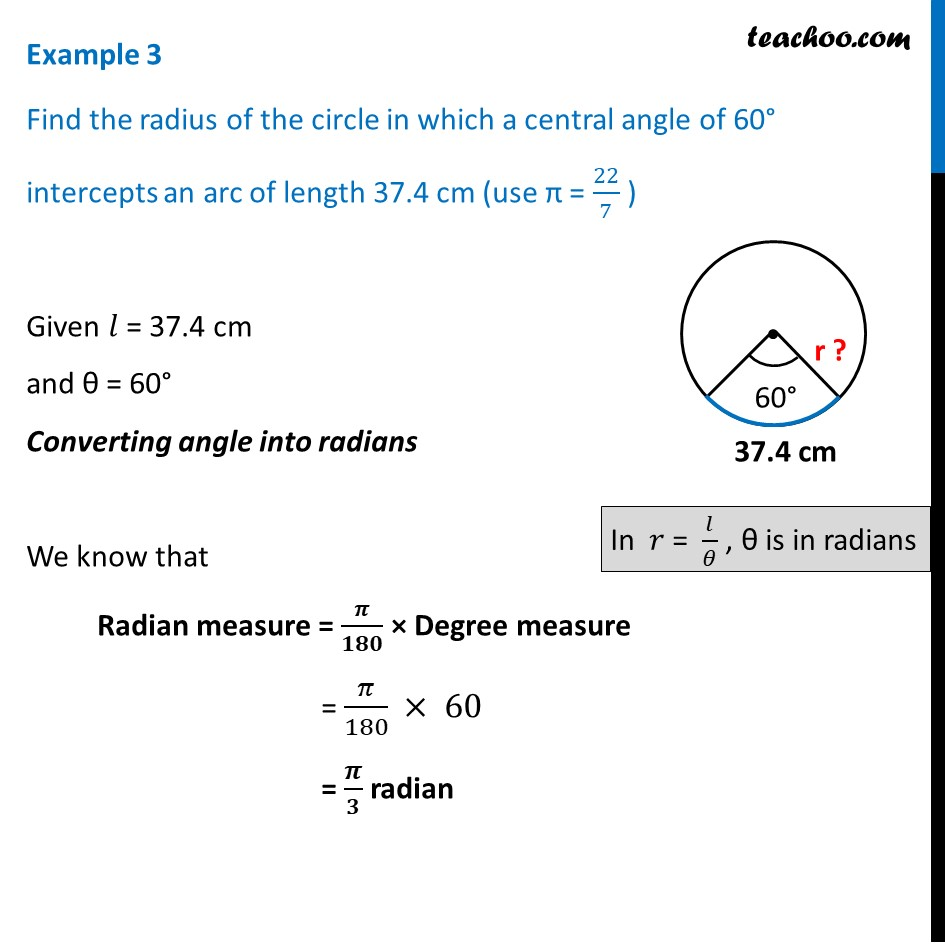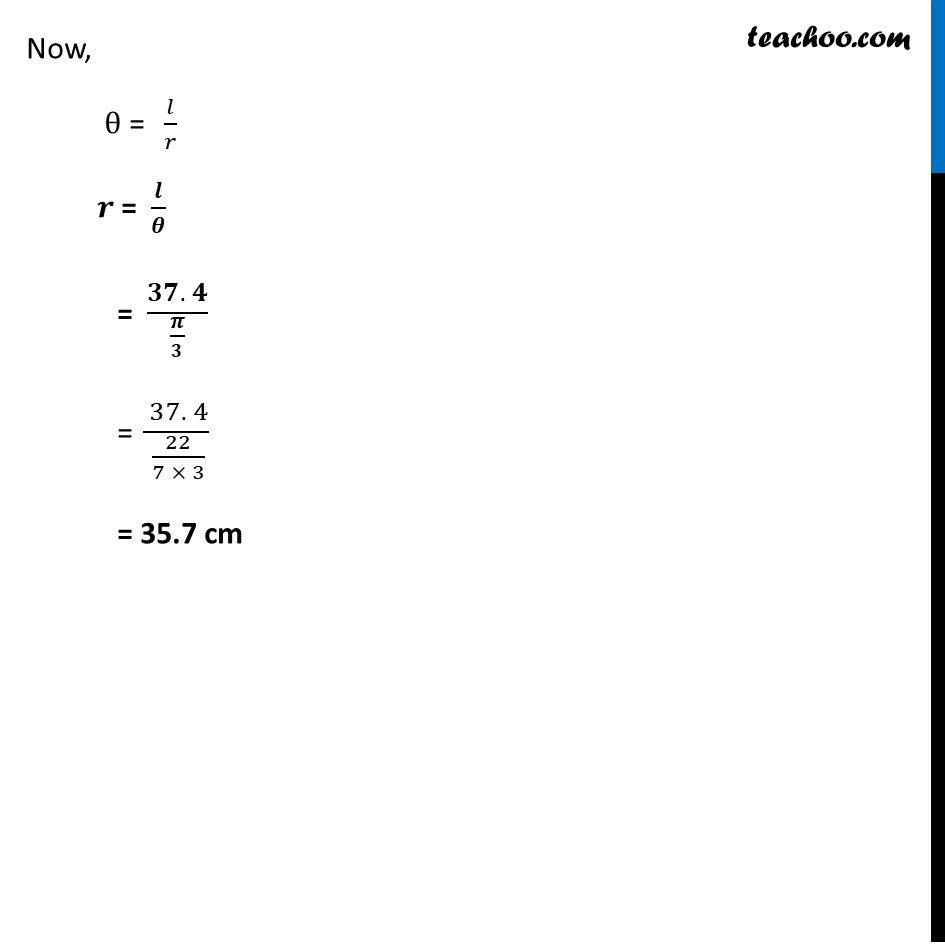Examples

Chapter 3 Class 11 Trigonometric Functions
Serial order wiseLearn in your speed, with individual attention - Teachoo Maths 1-on-1 Class

### Transcript

Example 3 Find the radius of the circle in which a central angle of 60° intercepts an arc of length 37.4 cm (use π = 22/7 ) Given 𝑙 = 37.4 cm and θ = 60° Converting angle into radians We know that Radian measure = 𝝅/𝟏𝟖𝟎 × Degree measure = 𝜋/180 × 60 = 𝝅/𝟑 radian Now, θ "= " 𝑙/𝑟 " " 𝒓 = 𝒍/𝜽 = (𝟑𝟕. 𝟒)/(𝝅/𝟑) = (37. 4)/(22/(7 × 3)) = 35.7 cm